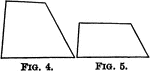### Equiangular Polygons

Illustration of a two polygons. Polygons may be mutually equiangular without being mutually equilateral.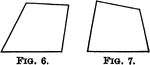### Equilateral Polygons

Illustration of two polygons. Polygons may be mutually equilateral without being mutually equiangular…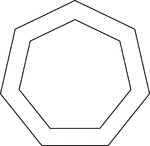### 2 Concentric Septagons/Heptagons

Illustration of 2 regular concentric heptagons/septagons.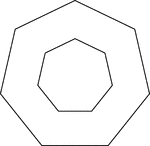### 2 Concentric Septagons/Heptagons

Illustration of 2 regular concentric heptagons/septagons.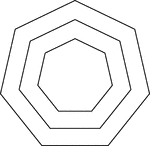### 3 Concentric Septagons/Heptagons

Illustration of 3 regular concentric heptagons/septagons that are equally spaced.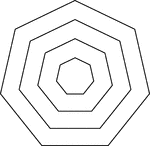### 4 Concentric Septagons/Heptagons

Illustration of 4 regular concentric heptagons/septagons that are equally spaced.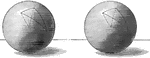### Equal Spheres With Triangles

Illustration of equal spheres with equilateral and equiangular triangles.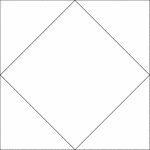### Square Inscribed In A Square

Illustration of a square inscribed in a square. The interior square is rotated 45° in relation to…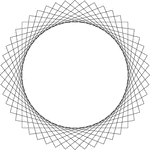### 12 Congruent Rotated Squares

Illustration of 12 congruent squares that have the same center. Each square has been rotated 7.5°…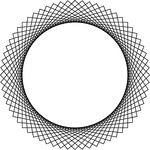### 16 Congruent Rotated Squares

Illustration of 16 congruent squares that have the same center. Each square has been rotated 5.625°…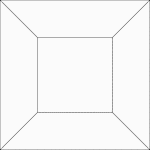### 2 Concentric Squares

Illustration of 2 concentric squares whose vertices are connected by line segments.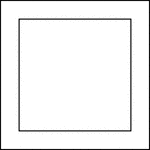### 2 Concentric Squares

Illustration of 2 concentric squares.### 2 Concentric Squares

Illustration of 2 concentric squares.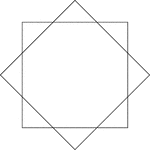### 2 Congruent Rotated Squares

Illustration of 2 congruent squares that have the same center. One square has been rotated 45° in…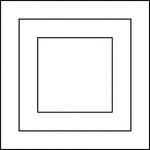### 3 Concentric Squares

Illustration of 3 concentric squares that are equally spaced.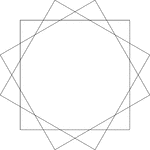### 3 Congruent Rotated Squares

Illustration of 3 congruent squares that have the same center. Each square has been rotated 30°…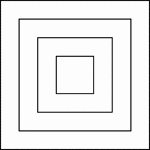### 4 Concentric Squares

Illustration of 4 concentric squares that are equally spaced.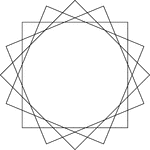### 4 Congruent Rotated Squares

Illustration of 4 congruent squares that have the same center. Each square has been rotated 22.5°…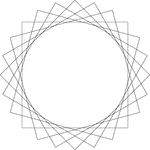### 6 Congruent Rotated Squares

Illustration of 6 congruent squares that have the same center. Each square has been rotated 15°…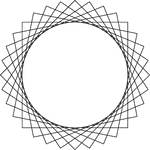### 8 Congruent Rotated Squares

Illustration of 8 congruent squares that have the same center. Each square has been rotated 11.25°…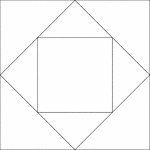### Squares Inscribed In Squares

Illustration of a square inscribed in a square that is inscribed in another square. Each successive…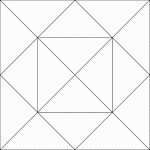### Squares Inscribed In Squares

Illustration of a square inscribed in a square that is inscribed in another square. Each successive…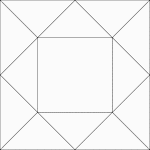### Squares Inscribed In Squares

Illustration of a square inscribed in a square that is inscribed in another square. Each successive…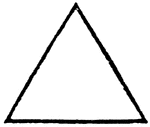### Equilateral triangle

"Having equal sides." — Williams, 1889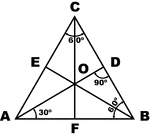### Equilateral Triangle

Sketch of equilateral triangle with bisectors, medians, and altitudes.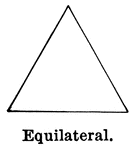### Equilateral Triangle

Illustration showing an equilateral triangle (one that has three sides equal).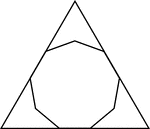### Nonagon Inscribed in a Triangle

Illustration of a regular nonagon inscribed in an equilateral triangle. This could also be described…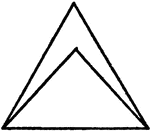### Equilateral And Isosceles Triangles

An illustration showing an isosceles triangle in an equilateral triangle.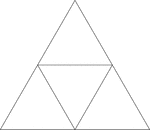### Equilateral Triangle Inscribed In An Equilateral Triangle

Illustration of an equilateral triangle inscribed in an equilateral triangle. The smaller triangle is…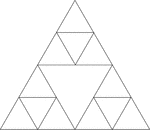### Equilateral Triangles Inscribed In An Equilateral Triangle

Illustration of an equilateral triangle inscribed in an equilateral triangle by joining the midpoints…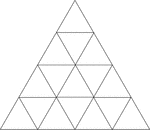### Equilateral Triangles Inscribed In Equilateral Triangles

Illustration of an equilateral triangle inscribed in an equilateral triangle by joining the midpoints…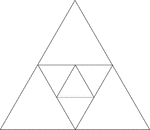### Equilateral Triangles Inscribed In Equilateral Triangles

Illustration of an equilateral triangle inscribed in an equilateral triangle by joining the midpoints…